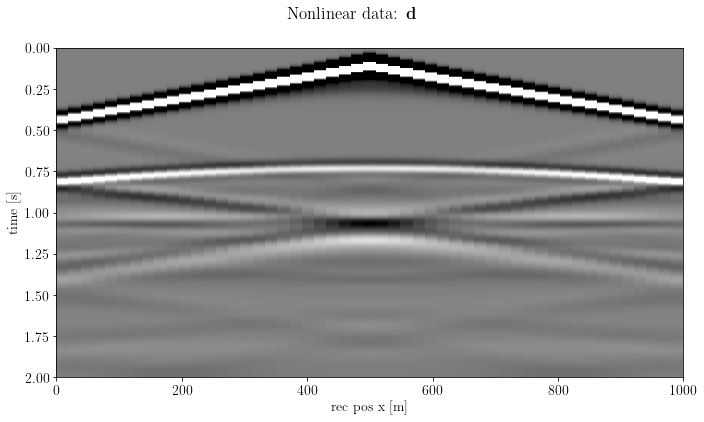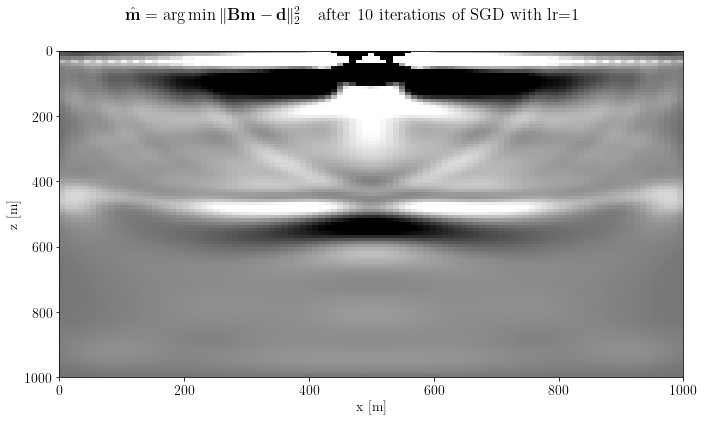Authors
Affiliations

# Least-Squares Reverse Time Migration through Devito and Automatic Differentiation¶

@Author: Francesco Picetti - picettifrancesco@gmail.com

In this tutorial, we put together torch's Automatic Differentiation abilities and the OccamyPy physical operators (well, actually Devito's) for RTM. Basically, we can write a standard pytorch optimization loop with the modeling provided by OccamyPy; the tricky part is to insert a linear operator (i.e., Born) in the AD graph.

To use this notebook, we need devito installed. On your occamypy-ready env, run

pip install --user git+https://github.com/devitocodes/devito.git

import torch
import occamypy as o
import born_devito as b

# Plotting
from matplotlib import rcParams
from mpl_toolkits.axes_grid1 import make_axes_locatable
import matplotlib.pyplot as plt
rcParams.update({
'image.cmap'     : 'gray',
'image.aspect'   : 'auto',
'image.interpolation': None,
'axes.grid'      : False,
'figure.figsize' : (10, 6),
'savefig.dpi'    : 300,
'axes.labelsize' : 14,
'axes.titlesize' : 16,
'font.size'      : 14,
'legend.fontsize': 14,
'xtick.labelsize': 14,
'ytick.labelsize': 14,
'text.usetex'    : True,
'font.family'    : 'serif',
'font.serif'     : 'Latin Modern Roman',
})
WARNING! DATAPATH not found. The folder /tmp will be used to write binary files

/nas/home/fpicetti/miniconda3/envs/occd/lib/python3.10/site-packages/dask_jobqueue/core.py:20: FutureWarning: tmpfile is deprecated and will be removed in a future release. Please use dask.utils.tmpfile instead.
from distributed.utils import tmpfile


## Setup¶

args = dict(
filter_sigma=(1, 1),
spacing=(10., 10.),  # meters
shape=(101, 101),    # samples
nbl=20,              # samples
src_x=,         # meters
src_depth=20.,       # meters
rec_depth=30.,       # meters
t0=0.,
tn=2000.,            # Simulation lasts 2 second (in ms)
f0=0.010,            # Source peak frequency is 10Hz (in kHz)
space_order=5,
kernel="OT2",
src_type='Ricker',
)
def unpad(model, nbl: int = args["nbl"]):
return model[nbl:-nbl, nbl:-nbl]

create hard model and migration model

model_true, model_smooth, water = b.create_models(args)
model = o.VectorNumpy(model_smooth.vp.data.__array__())
model.ax_info = [
o.AxInfo(model_smooth.vp.shape, model_smooth.origin - model_smooth.nbl * model_smooth.spacing,
model_smooth.spacing, "x [m]"),
o.AxInfo(model_smooth.vp.shape, model_smooth.origin - model_smooth.nbl * model_smooth.spacing,
model_smooth.spacing, "z [m]")]
model_extent = [0., 1000., 1000., 0.]

Define acquisition geometry

rec = b.build_rec_coordinates(model_true, args)
src = [b.build_src_coordinates(x, args["src_depth"]) for x in args["src_x"]]

### create the common shot gather (CSG) data¶

d = o.VectorTorch(b.propagate_shots(model=model_true, src_pos=src, rec_pos=rec, param=args))
assert d[:].requires_grad is False
fig, axs = plt.subplots(1, 1, sharey=True)
axs.imshow(d.plot(), clim=o.plot.clim(d[:]),
extent=[d.ax_info.o, d.ax_info.last, d.ax_info.last, d.ax_info.o])
axs.set_xlabel(d.ax_info.l)
axs.set_ylabel(d.ax_info.l)
fig.suptitle(r"Nonlinear data: $\mathbf{d}$")
plt.tight_layout()
plt.show()## Instantiate the Born operator...¶

B = b.BornSingleSource(velocity=model_smooth, src_pos=src, rec_pos=rec, args=args)

### ... and cast it to an Autograd function¶

B_ = o.torch.AutogradFunction(B)

instantiate the model as a "learnable" vector, i.e., a new kind of VectorTorch that encapsulate a tensor attached to the AD graph

m = o.torch.VectorAD(torch.zeros(model.shape), ax_info=model.ax_info)

m.setDevice(None)

assert m[:].requires_grad is True

instantiate the optimizer that will work on the model

opt = torch.optim.SGD([m.getNdArray()], lr=1)

define the objective function terms: the mean squared error...

mse = torch.nn.MSELoss().to(m.device)

and more complex terms (the TV, for example) that can be based on OccamyPy operators!

G = o.torch.AutogradFunction(o.FirstDerivative(model, axis=0))
l1 = torch.nn.L1Loss().to(m.device)
tv = lambda x: l1(G(x), torch.zeros_like(x))

## Optimization loop¶

epochs = 10
for epoch in range(epochs):

# modeling
d_ = B_(m)

# note: things can be a lot complicated! Deep Priors, CNN-based denoisers, ecc ecc

# objective function
loss = mse(d_.float(), d[:]) + 0.1 * tv(m.getNdArray())

# model update
loss.backward()
opt.step()

# log
print("Epoch %s, obj = %.2e" % (str(epoch).zfill(3), loss))
Epoch 000, obj = 1.44e+00
Epoch 001, obj = 1.02e+00
Epoch 002, obj = 8.32e-01
Epoch 003, obj = 7.27e-01
Epoch 004, obj = 6.63e-01
Epoch 005, obj = 6.20e-01
Epoch 006, obj = 5.89e-01
Epoch 007, obj = 5.66e-01
Epoch 008, obj = 5.47e-01
Epoch 009, obj = 5.32e-01

fig, axs = plt.subplots(1, 1, sharey=True)
fig.suptitle(r"$\hat{\mathbf{m}} = \arg \min \Vert \mathbf{B}\mathbf{m} - \mathbf{d} \Vert_2^2 \quad$" + f"after {epochs} iterations of SGD with lr={opt.param_groups['lr']}")
plt.show()In principle, an AutogradFunction can be casted also for Dask-distributed operators. However, distributing a shot to each workers (as done in the previous tutorial) will require the objective function to be computed on different workers, too.